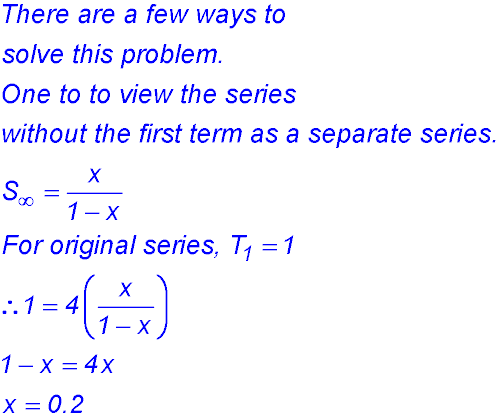Dr. J's Maths.com
Where the techniques of Maths
are explained in simple terms.

Sequences & Series - Geometric - Limiting sum.
Test Yourself 1 - Solutions.

 Using the limiting sum relationship. 1.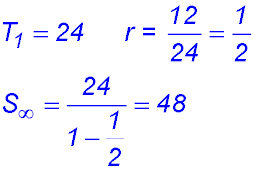2.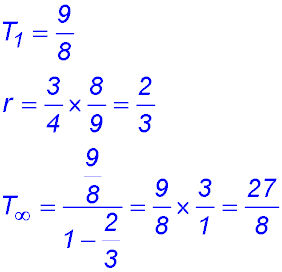Find r. 3.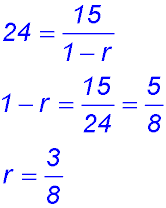4.5. As we do not know the first term, we write T1× r = 5 so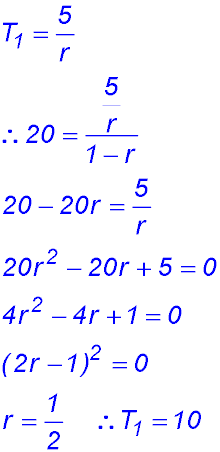6.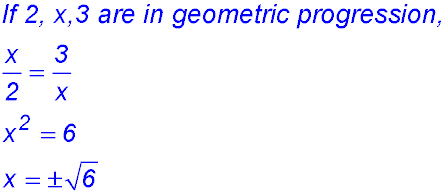7.8.Conditions for a limiting sum 9.10. (i)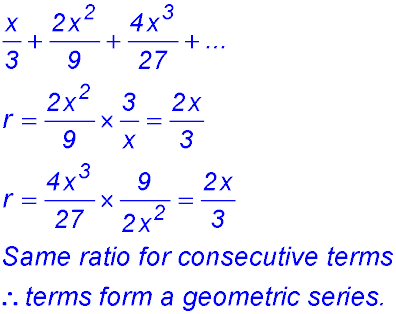(ii)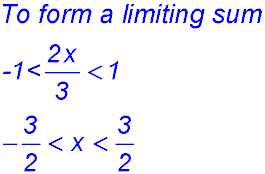(iii)11.12.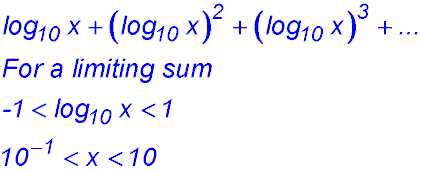13.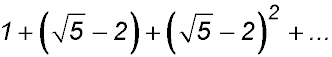(i)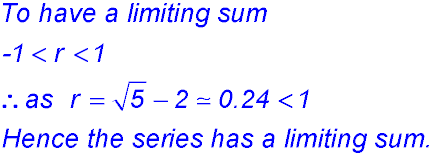(ii)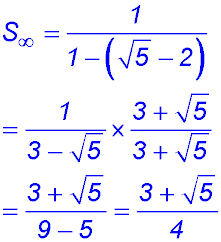14.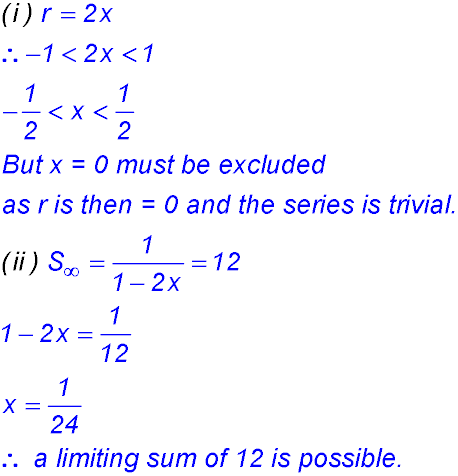15. (i) -1 < b < 1.(ii)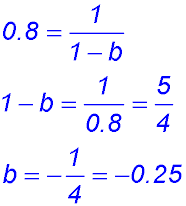16. (i)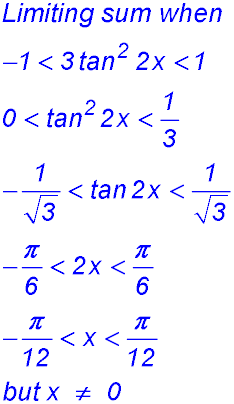(ii) Recurring decimals. 17.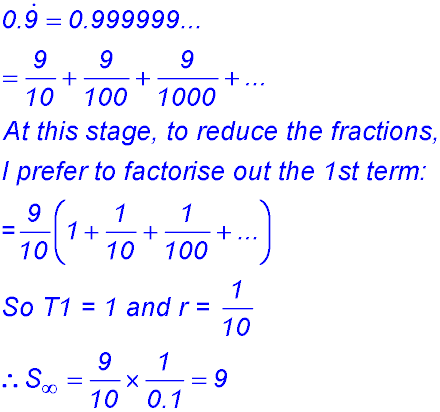18.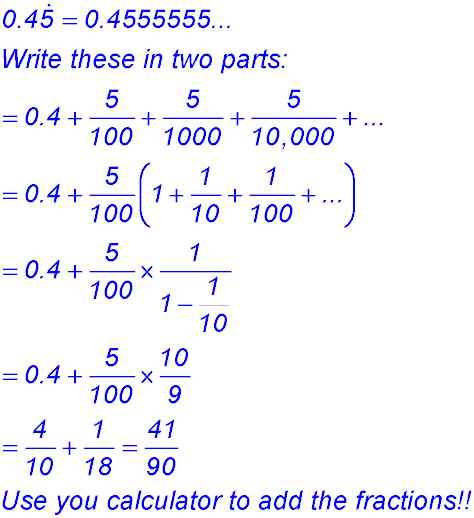19.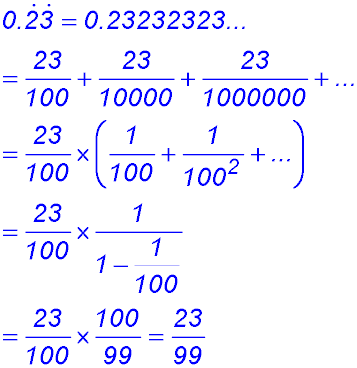20.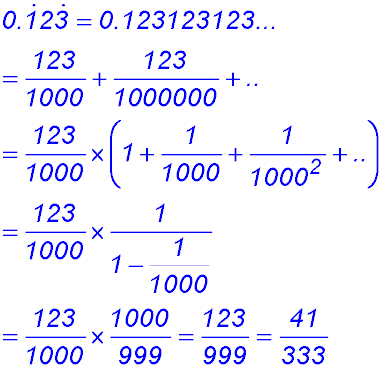Mixture 21.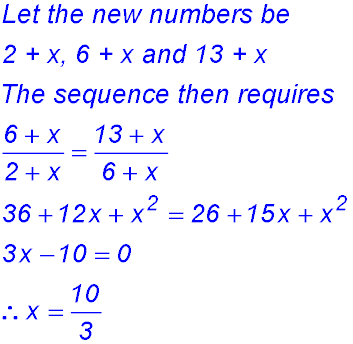22.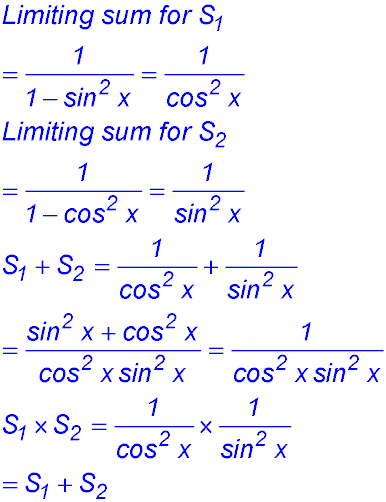Applied questions. 23. (i) In the 1st year after Jim planted the tree, it grew (228 cm - 120 cm) = 108 cm. This amount of growth is the same as 120 cm × 90%. Note we are measuring the growth each year NOT the height of the tree at the end of the three years. That would require the use of the "sum formula". Use the 108 cm growth in the first year after planting as the first term. In the second year, the tree grew 108 × 90% = 97.20 cm. In the third year, the tree grew by 108 × (90%)2= 87.48 cm. (ii) To calculate the height after 5 years, we sum the increases in height and add that overall increase to the original height of 120 cm.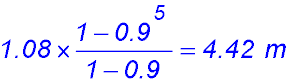Add this total change in height to the original height at planting (1.20 m) gives the height of the tree as 5.62 m after 5 years (iii) To "ever reach" tells us we need a limiting sum So the total change in height should be 12 m - 1.20 m = 10.8 m.So final height is 1.20 m + 10.8 m = 12m. Alternatively, the tree growth coud be viewed as the series: 120 + (120×0.9)+ (120×0.92) + (120×0.923) + ... So a first term of 120 and an r = 0.9 giving a limiting sum of 12 m. 24.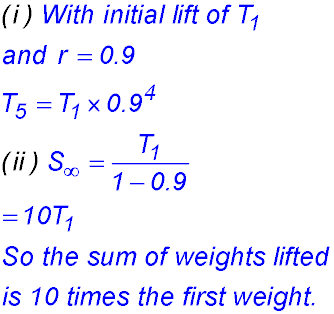25.